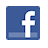Games
Problems
Go Pro!

# System with Radical

Pro Problems > Math > Algebra > Equations > Systems of Equations > Non-Linear

## System with Radical

The sum of two numbers is seven times the difference between three times the second number and twice the first number. If the second number is subtracted from the first, the result is the square root of the first. Find all possible values for the first number.

Presentation mode
Problem by Mr. H

## Solution

In order to make it feasible for teachers to use these problems in their classwork, no solutions are publicly visible, so students cannot simply look up the answers. If you would like to view the solutions to these problems, you must have a Virtual Classroom subscription.Assign this problem
Click here to assign this problem to your students.

## Similar Problems

### Mary and Laura's Cookies

Mary and Laura Ingalls each receive a cookie. Because they are thoughtful children, they want to share with their little sister Carrie. Because their math skills aren't very advanced, they each eat half of a cookie, but then realize that leaves a full cookie for Carrie.

How much should each girl eat in order to share equally among the three sisters?

### X and Y System

Find all ordered pairs (x,y) which solve the following non-linear system of equations.

x(x - 2y) - 4 = 2y(x - 2y)

x + 2y = 10

### Quadratic System

Solve for m and n.

(m + n)2 - 10(m + n) + 24  = 0

(m - n)2 + 6(m - n) + 8 = 0

### Cubic and Linear

Find all ordered pairs (x,y) which solve the following system of equations:

x3 + 12xy2 = 7x2y

x + y = 20

### Sum of X and Y

Find the sum of x and y if x and y are positive numbers such that x2 + 3xy + y2 = 424 and xy = 100

### Linear and Quadratic

Find all ordered pairs (x, y) such that:

3x - y = 10

x2 + 8x - y2 + 3y = 17

### Quadratic System

Find all ordered pairs (x, y) such that the following two equations are true:

x2 - 4y2 = 108

x = 18 - 2y

### X and Y Quadratics

Find the sum of x and y, if the following are true:

(x + 2)(x - 1) = (y - 12)(y + 3)

(x + 1)(x + 3) = (y - 5)(y - 7)

### System with a Product

Find all ordered pairs (x, y) such that

2x + xy + y = 18

x - y = 2

### Product of X and Y

For the ordered pair (x,y) the product of x and y is 108. If x + 2y = 30, find all possible ordered pairs (x,y).

To Sum It Up, One Equation, Two Variables, Sum and Product System

# Featured Games on This Site

Match color, font, and letter in this strategy game
Trap all the dots in this problem-solving puzzle

# Blogs on This SiteReviews and book lists - books we love!The site administrator fields questions from visitors.Like us on Facebook to get updates about new resources